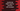# JavaScript Math expm1() function## JavaScript Math expm1() function :

expm1() is defined in Math. It is a static method and you can call it directly like Math.expm1(). It takes one number as the argument and returns e^n - 1, where n is the provided number. That means, its value is equal to Math.exp(n) - 1.

## Example of Math.expm1 :

Let’s consider the below example :

``````console.log(Math.expm1(0));
console.log(Math.expm1(1));
console.log(Math.expm1(Math.E));
console.log(Math.expm1(-10));``````

Run it and it will print the below output :

``````0
1.718281828459045
14.154262241479262
-0.9999546000702375``````

## Math.expm1() and Math.exp() :

Let’s compare Math.expm1 and Math.exp :

``````console.log(`\${Math.expm1(0)} = \${Math.exp(0) - 1}` );
console.log(`\${Math.expm1(1)} = \${Math.exp(1) - 1}` );
console.log(`\${Math.expm1(Math.E)} = \${Math.exp(Math.E) - 1}` );
console.log(`\${Math.expm1(-10)} = \${Math.exp(-10) - 1}` );``````

It will print :

``````0 = 0
1.718281828459045 = 1.718281828459045
14.154262241479262 = 14.154262241479262
-0.9999546000702375 = -0.9999546000702375``````

So, Math.expm1 is equal to Math.exp minus 1 for a number.

## Math.expm1 with different types :

For the below example :

``````console.log(Math.expm1("2"));
console.log(Math.expm1(2.4));
console.log(Math.expm1("2.4"));
console.log(Math.expm1(null));
console.log(Math.expm1(undefined));
console.log(Math.expm1());
console.log(Math.expm1("s"));``````

It will print :

``````6.38905609893065
10.023176380641601
10.023176380641601
0
NaN
NaN
NaN``````

For null, it returns 0. For other values, it tries to convert to a number. If it converts, it calculates for that value, else returns NaN.